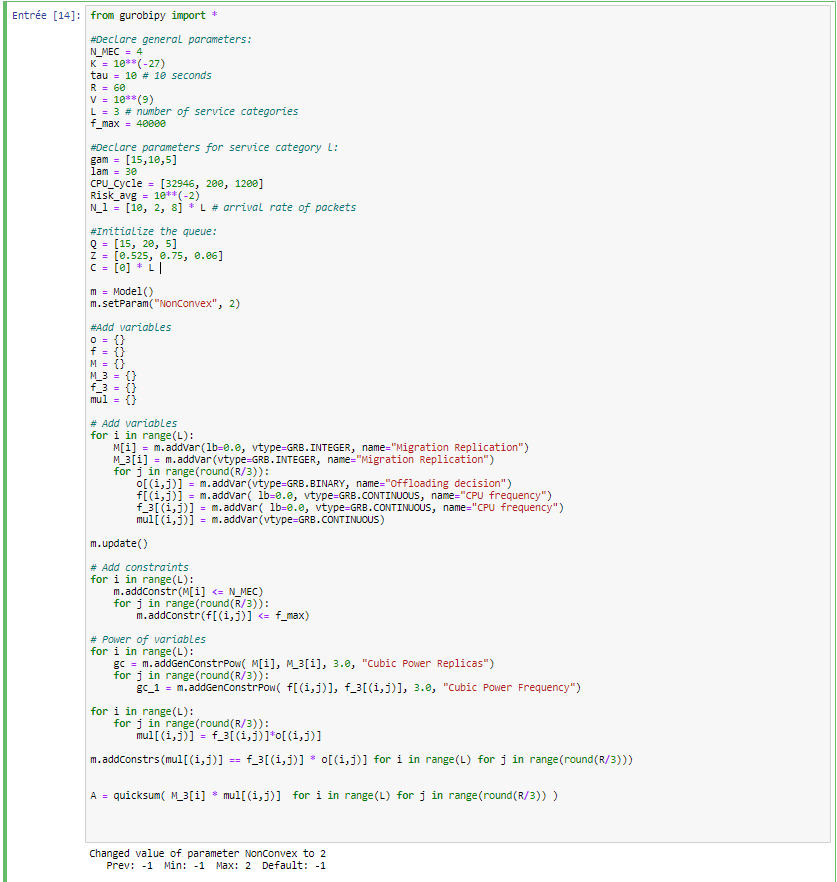•Gurobi Staff

Hi Ibtissam,

In the line

quicksum( M_3[i] * f_3[(i,j)] * o[(i,j)] for i in range(L) for j in range(R) )

you are trying to add products of three variables. This is not supported. You can only have products of at most two, i.e., quadratic terms.

You can make the other approach work, but you have to define mul as new variables and then make

mul[(i,j)] = f_3[(i,j)] * o[(i,j)

constraints in the model, i.e.,

m.addConstrs(mul[(i,j)] == f_3[(i,j)] * o[(i,j)] for i in range(L) for j in range(R))

Please note that with (all the bilinear and power constraints) the resulting model may be hard to solve and the result could have approximation/rounding errors.

•Hello Silke,

This is part of what my code looks like :I'm aware that the problem can be complex to solve, but I'd like to see how it plays out.

Regards,

Ibtissam

•Gurobi Staff

Hi Ibtissam,

Could you try without

mul[(i,j)] = f_3[(i,j)]*o[(i,j)] ?

Best regards,
Silke

•Hello Silke,

Indeed it is working !

Thank you.

Best Regards,

Ibtissam